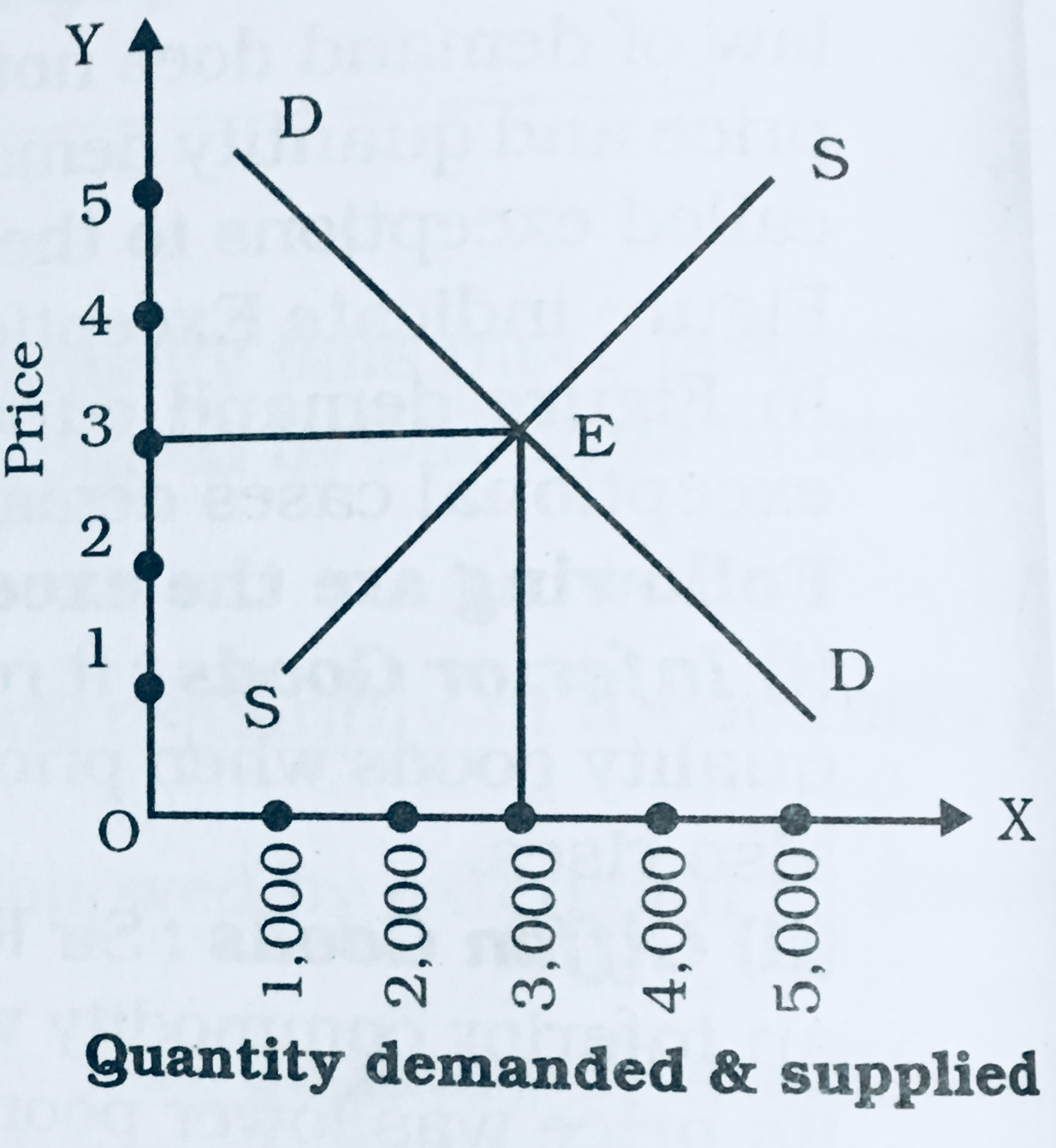SSC BOARD PAPERS IMPORTANT TOPICS COVERED FOR BOARD EXAM 2024

### What is perfect competition? Explain price determination under perfect competition.

What is perfect competition? Explain price determination under perfect competition.

Perfect competition is a market situation where there are large number of buyers and seller. Buying and selling homogenous products at a single uniform price.

Conditions of perfect competition are
(a)   Large number of buyers and sellers
(b)   Homogeneous product
(c)    Uniform price
(d)   Free entry and Exist
(e)    Perfect Knowledge
(f)     Perfect mobility of production factors.
(g)   Absence if transport cost
(h)   Absence of Government interference

Price Determination under Perfect Competition
Equilibrium price: Equilibrium price is the price at which quantity demanded is equal to quantity supplied. The price of the product under perfect competition, is influenced by both buyers and sellers and equilibrium price is determined by the interaction of demand and supply forces. According to Marshall, demand and supply are like two blades of a pair of scissors. Just as cutting of cloth is not possible with the use of one blade, the equilibrium price of a commodity cannot be determined, either by the forces of demand or by supply alone. Both together determine the price.
We can study this with the help of the following table and graph.

Demand and Supply Schedule
 Price (Rs) Per Unit Quantity Demanded (Units) Quantity Supplied (Units) 5 100 500 4 200 400 3 300 300 2 400 200 1 500 100

Above table shows the effect of price on market demand and market supply. The table shows that when price of the commodity is Rs 5, quantity demanded is 100 units and quantity supplied is 500 units. Since supply is more than demand, price falls to Rs 4/- and Rs 3/-, respectively and quantity demanded extends to 200 and 300 units whereas supply contracted to 400 and 300 units, respectively. It is seen that quantity demanded and that quantity supplied both are equal at Rs. 3 where quantity demanded is 300 units whereas quantity supplied is 300 units. Thus, Rs. 3 will be an equilibirim price.

If further price falls to Rs. 2 and Rs,1, quantity demanded will expand to 400 and 500 units, respectively and quantity supplied will contract to 200 and 100 units respectively. Since demand is more than supply, competition among buyers will increase and price will rise up to Rs. 2 and Rs. 3.  Thus equilibrium price will be `Rs. 3 per unit because demand and supply both are equal at this price.In the above diagram OX axis indicate quantity demanded and supplied and OY axis indicate price. Demand Curve DD intersect supply curve SS at E point where Rs. 3 is an equilibrium price and 300 is an equilibrium quantity thus under perfect competition an equilibrium price is determined by itersection of demand and supply curve.